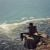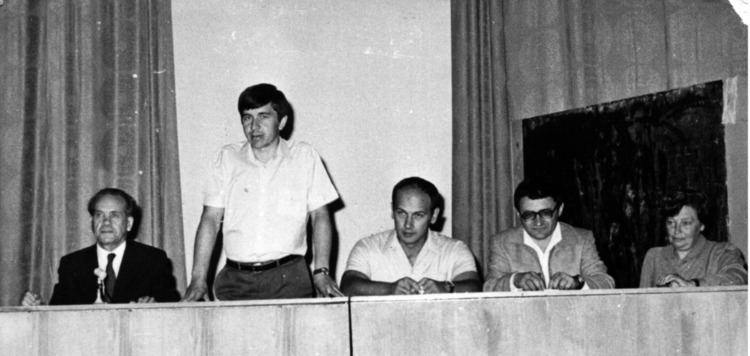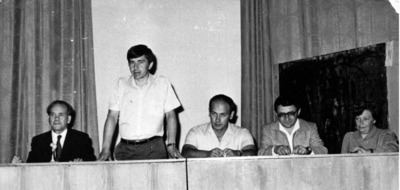# Aizik Volpert

Updated on
Covid-19
 Name  Aizik Volpert Role  MathematicianBorn  June 5, 1923 Kharkov (1923-06-05) Institutions  Lviv University Lviv Industrial Forestry Institute TechnionAlma mater  Lviv University Moscow UniversityKnown for  Functions of bounded variation index theory partial differential equations parabolic partial differential equations mathematical chemistryDied  January 2006, Haifa, IsraelEducation  Moscow State University, Lviv University

Aizik Isaakovich Vol'pert (Russian: Айзик Исаакович Вольперт) (5 June 1923 – January 2006) (the family name is also transliterated as Volpert or Wolpert) was a Soviet and Israeli mathematician and chemical engineer working in partial differential equations, functions of bounded variation and chemical kinetics.

## ContentsVol'pert graduated from Lviv University in 1951, earning the candidate of science degree and the docent title respectively in 1954 and 1956 from the same university: from 1951 on he worked at the Lviv Industrial Forestry Institute. In 1961 he became senior research fellow while 1962 he earned the "doktor nauk" degree from Moscow State University. In the 1970s–1980s A. I. Volpert became one of the leaders of the Russian Mathematical Chemistry scientific community. He finally joined Technion’s Faculty of Mathematics in 1993, doing is Aliyah in 1994.

## Index theory and elliptic boundary problems

Vol'pert developed an effective algorithm for calculating the index of an elliptic problem before the Atiyah-Singer index theorem appeared: He was also the first to show that the index of a singular matrix operator can be different from zero.

## Functions of bounded variation

He was one of the leading contributors to the theory of BV-functions: he introduced the concept of functional superposition, which enabled him to construct a calculus for such functions and applying it in the theory of partial differential equations. Precisely, given a continuously differentiable function f : ℝp → ℝ and a function of bounded variation u(x) = (u1(x),...,up(x)) with x ∈ ℝn and n ≥ 1, he proves that fu(x) = f(u(x)) is again a function of bounded variation and the following chain rule formula holds:

f ( u ( x ) ) x i = k = 1 p f ¯ ( u ( x ) ) u k u k ( x ) x i i = 1 , , n

where f(u(x)) is the already cited functional superposition of f and u. By using his results, it is easy to prove that functions of bounded variation form an algebra of discontinuous functions: in particular, using his calculus for n = 1, it is possible to define the product H ⋅ δ of the Heaviside step function H(x) and the Dirac distribution δ(x) in one variable.

## Chemical kinetics

His work on chemical kinetics and chemical engineering led him to define and study differential equations on graphs.

## Selected publications

• Hudjaev, Sergei Ivanovich; Vol'pert, Aizik Isaakovich (1985), Analysis in classes of discontinuous functions and equations of mathematical physics, Mechanics: analysis, 8, Dordrecht–Boston–Lancaster: Martinus Nijhoff Publishers, pp. xviii+678, ISBN 90-247-3109-7, MR 0785938, Zbl 0564.46025 . One of the best books about BV-functions and their application to problems of mathematical physics, particularly chemical kinetics.
• Vol'pert, Aizik Isaakovich (1967), Пространства BV и квазилинейные уравнени, Matematicheskii Sbornik, (N.S.) (in Russian), 73(115) (2): 255–302, MR 216338, Zbl 0168.07402 . A seminal paper where Caccioppoli sets and BV functions are thoroughly studied and the concept of functional superposition is introduced and applied to the theory of partial differential equations: it was also translated as "Spaces BV and quasi-linear equations", Mathematics of the USSR-Sbornik, 2 (2): 225–267, 1967, MR 216338, Zbl 0168.07402, doi:10.1070/SM1967v002n02ABEH002340, (Subscription required (help)) .
• Vol'pert, Aizik Isaakovich (1972), Дифференциальные уравнения на графах, Matematicheskii Sbornik, (N.S.) (in Russian), 88(130) (4(8)): 578–588, MR 316796, Zbl 0242.35015 , translated in English as "Differential equations on graphs", Mathematics of the USSR-Sbornik, 17 (4): 571–582, 1972, Zbl 0255.35013, doi:10.1070/SM1972v017n04ABEH001603, (Subscription required (help)) .
• Vasiliev, V. M.; Volpert, A. I.; Hudiaev, S. I. (1973), "On the method of quasi-stationary concentrations for chemical kinetics equations", Журнал вычислительной математики и математической физики (in Russian), 13 (3): 683–697 .
• Vol'pert, A. I. (1976), "Qualitative methods of investigation of equations of chemical kinetics", Preprint (in Russian), Institute of Chemical Physics, Chernogolovka .
• Vol'pert, V. A.; Vol'pert, A. I.; Merzhanov, A. G. (1982), "Application of the theory of bifurcations in study of the spinning combustion waves", Doklady Akademii Nauk SSSR (in Russian), 262 (3): 642–645 .
• Vol'pert, V. A.; Vol'pert, A. I.; Merzhanov, A. G. (1982b), "Analysis of nonunidimensional combustion modes by bifurcation theory methods", Doklady Akademii Nauk SSSR (in Russian), 263 (4): 918–921 .
• Vol'pert, V. A.; Vol'pert, A. I.; Merzhanov, A. G. (1983), "Application of the theory of bifurcations to the study of unsteady regimes of combustion", Fizika Goreniia i Vzryva (in Russian), 19: 69–72 , translated in English as "Application of the theory of bifurcations to the investigation of nonstationary combustion regimes", Combustion, Explosion, and Shock Waves, 19 (4): 435–438, 1983, doi:10.1007/BF00783642, (Subscription required (help)) .
• Vol'pert, V. A.; Vol'pert, A. I. (1989), "Existence and stability of traveling waves in chemical kinetics", Dynamics of Chemical and Biological Systems (in Russian), Novosibirsk: Nauka, pp. 56–131 .
• Vol'pert, Aizik I.; Vol'pert, Vitaly A.; Vol'pert, Vladimir A. (1994), Traveling Wave Solutions of Parabolic Systems, Translations of Mathematical Monographs, 140, Providence, R.I.: American Mathematical Society, pp. xii+448, ISBN 0-8218-3393-6, MR 1297766, Zbl 1001.35060 .
• Vol'pert, A. I. (1996), "Propagation of Waves Described by Nonlinear Parabolic Equations (a commentary on article 6)", in Oleinik, O. A., I. G. Petrovsky Selected works. Part II: Differential equations and probability theory, Classics of Soviet Mathematics, 5 (part 2), Amsterdam: Gordon and Breach Publishers, pp. 364–399, ISBN 2-88124-979-5, MR 1677648, Zbl 0948.01043 .
• Vol'pert, V. A.; Vol'pert, A. I. (1998), "Convective instability of reaction fronts: linear stability analysis", European Journal of Applied Mathematics, 9 (5): 507–525, MR 1662311, Zbl 0918.76027, (Subscription required (help)) .
• ## References

Similar Topics
The Toy Shoppe
Craig Brackins
Robert Neild
Topics